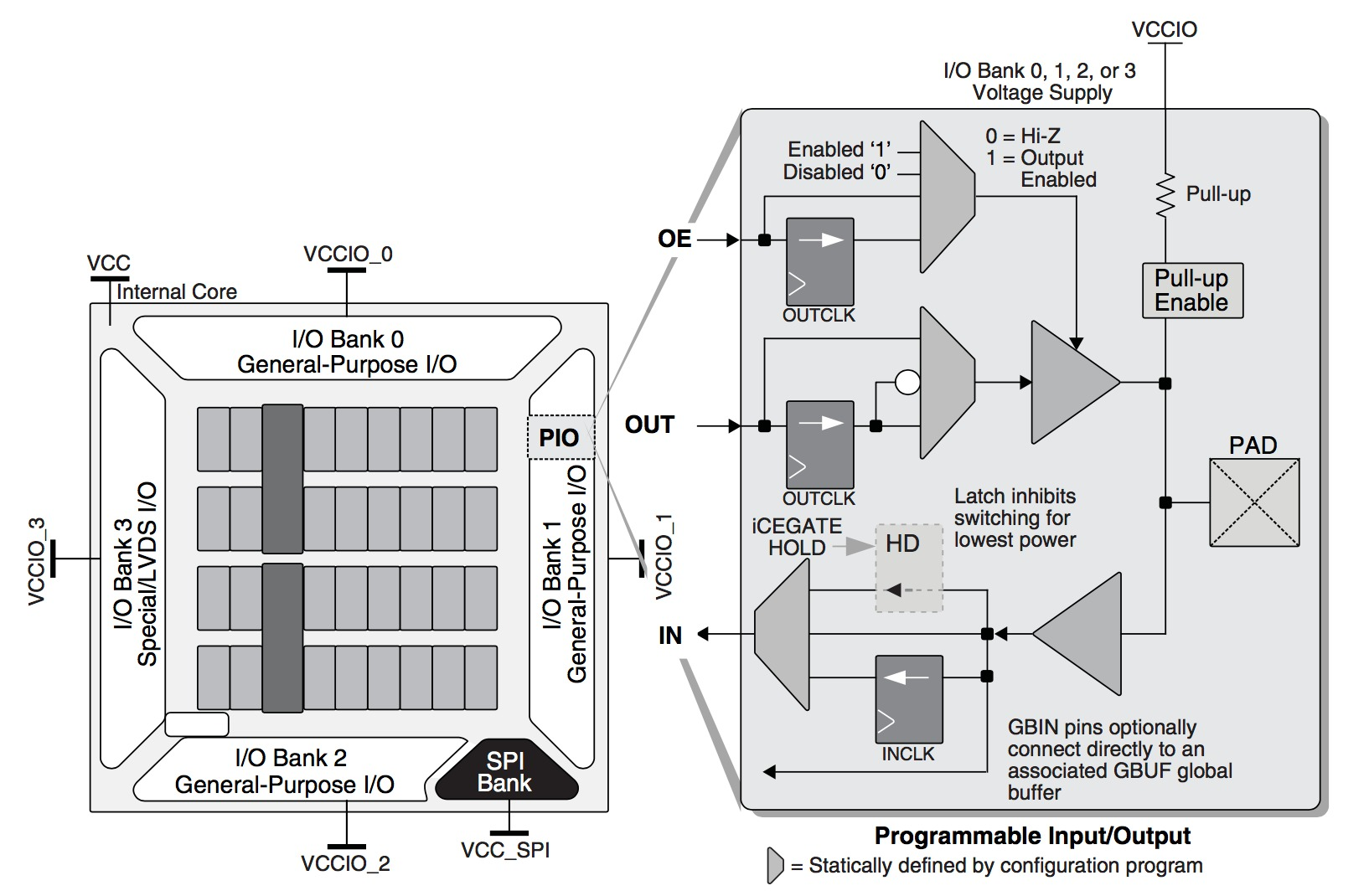# Lattice ICE40

These circuits are the low-level primitives for the Lattice ICE40 FPGAs, originally designed by Silicon Blue (hence, the prefix `SB_`).

Detailed documentation on these primitives is available in the Lattice iCE Technology Library and the Lattice iCE40 LP/HX Family Datasheet.

## Programmable Logic Block

The programmable logic block consists of a vertical column of 8 logic cells. Each logic cell containts three components, a 4-bit lookup table (LUT4), a Carry unit, and a D-flip-flop (DFF)..### LUTS

The combinational logic primitive is a 4-bit LUT.

``````# SB_LUT4 :: I0:In(Bit), I1:In(Bit), I2:In(Bit), I3:In(Bit), O:Out(Bit)
SB_LUT4(LUT_INIT=int16))
``````

The LUT is initialized by setting the parameter INIT to a 16-bit integer value. The output for a given input is equal to the bit at the position corresponding to the input value.

### Carry

``````# SB_CARRY() :: I0:In(Bit), I1:In(Bit), CI:In(Bit), CO:Out(Bit)
SB_CARRY()
``````

This functional unit computes the carry out given 3 inputs, including the carry in which must come from the previous logic cell in the vertical column.

``````CO = (I0&I1)|(I1&CI)|(CI&I0)
``````

### Flip-Flops

The ice40 DFF flip-flop can be configured in many different ways.

``````SB_DFF(init=0, has_ce=False, has_reset=False, has_set=False, edge=True, sync=True)
``````

The input can be stored in the flip-flop on the rising (edge=True) or falling (edge=False) edge. The flip-flop can optionally contain a clock enable. The flip-flop can be configured to have a reset or set input, but not both. The reset and set can be configured to be either synchronous (sync=True) or asynchroous (sync=False). The DFFs are always initialized to 0.

``````# DFF w/ Clock enable
SB_DFFE() :: C:In(Bit), E:In(Bit), D:In(Bit), Q:Out(Bit)
# DFF w/ Synchronous Reset
SB_DFFSR() :: C:In(Bit), R:In(Bit), D:In(Bit), Q:Out(Bit)
# DFF w/ Asynchronous Reset
SB_DFFR() :: C:In(Bit), R:In(Bit), D:In(Bit), Q:Out(Bit)
# DFF w/ Synchronous Set
SB_DFFSS() :: C:In(Bit), S:In(Bit), D:In(Bit), Q:Out(Bit)
# DFF w/ Asynchronous Set
SB_DFFS() :: C:In(Bit), S:In(Bit), D:In(Bit), Q:Out(Bit)
# DFF w/ Synchronous Reset, Clock enable
SB_DFFESR() :: C:In(Bit), E:In(Bit), R:In(Bit), D:In(Bit), Q:Out(Bit)
# DFF w/ Asynchronous Reset, Clock enable
SB_DFFER() :: C:In(Bit), E:In(Bit), R:In(Bit), D:In(Bit), Q:Out(Bit)
# DFF w/ Synchronous Set, Clock enable
SB_DFFESS() :: C:In(Bit), E:In(Bit), S:In(Bit), D:In(Bit), Q:Out(Bit)
# DFF w/ Asynchronous Set, Clock enable
SB_DFFES() :: C:In(Bit), E:In(Bit), S:In(Bit), D:In(Bit), Q:Out(Bit)
``````

## MemoryThe memory blocks on the ice40 are 4 kilo-bits, and instanced using the following function.

``````# SB_RAM40_4K() ::
#    RCLKE:In(Bit),       # Read Clock Enable
#    WCLK:In(Bit),        # Write Clock
#    WCLKE:In(Bit),       # Write Clock Enable
#    WE:In(Bit),          # Write Enable
#    WDATA:In(Bits(16))   # Write Data
SB_RAM40_4K() ::
``````

There is a utility function `RAMB` which configures the memory as a RAM, and another `ROMB` that configures it as a ROM. This memory is initialized with the contents of `rom` (for `ROMB`) or `ram` (for `RAMB`). The aspect ratio (height x width) of the memory depends on the length of the array `rom` or `ram`. The following aspect ratios are allowed: 2048x2, 1024x4, 512x8, 256x16.

``````# RADDR: In(Bits(height),
# RDATA, In(Bits(width),
# RCLK,  In(Bit),
# RCLKE, In(Bit),
# RE,    In(Bit)
# WDATA, In(Bits(width),
# WCLK,  In(Bit),
# WCLKE, In(Bit),
# WE,    In(Bit)
ROMB(height, width, ram=None):
``````
``````# RADDR: In(Bits(height),
# RDATA, In(Bits(width),
# RCLK,  In(Bit),
# RCLKE, In(Bit),
# RE,    In(Bit)
ROMB(height, width, rom=None):
``````

## IO``````# SB_IO() ::
#    CLOCK_ENABLE:In(Bit),
#    INPUT_CLOCK':In(Bit),
#    OUTPUT_CLOCK':In(Bit),
#    OUTPUT_ENABLE':In(Bit),
#    LATCH_INPUT_VALUE':In(Bit),
#    D_IN_0':In(Bit), # rising
#    D_IN_1':In(Bit), # falling
#    PACKAGE_PIN: InOut(Bit)
#    D_OUT_0':Out(Bit), # rising
#    D_OUT_1':Bit, # falling
#    PACKAGE_PIN: Bit # inout
SB_IO(PIN_TYPE=int6)
``````

This primitive is controlled using the parameter `PIN_TYPE`.

## PLL

A PLL can be created using the following useful function.

``````# SB_PLL :: I, In(Bit), O, Out(Bit)
SB_PLL( freqout, freqin=12000000 ):
``````

Here, `I` is the reference clock and `O` is the new clock signsl.

This function configures the low-level primitive `SB_PLL40_CORE`.

``````# SB_PLL40_CORE ::
#            "REFERENCECLK", In(Bit),
#            "RESETB", In(Bit),
#            "BYPASS", In(Bit),
#            "PLLOUTCORE", Out(Bit),
#            "PLLOUTGLOBAL", Out(Bit)
``````